## Orthogonal projection on a Line Segment and its True Length

### True length of a line segment

 If a line segment AB doesn't lie on a ray of projection, then its horizontal and vertical projections are line segments A'B' and  A''B''.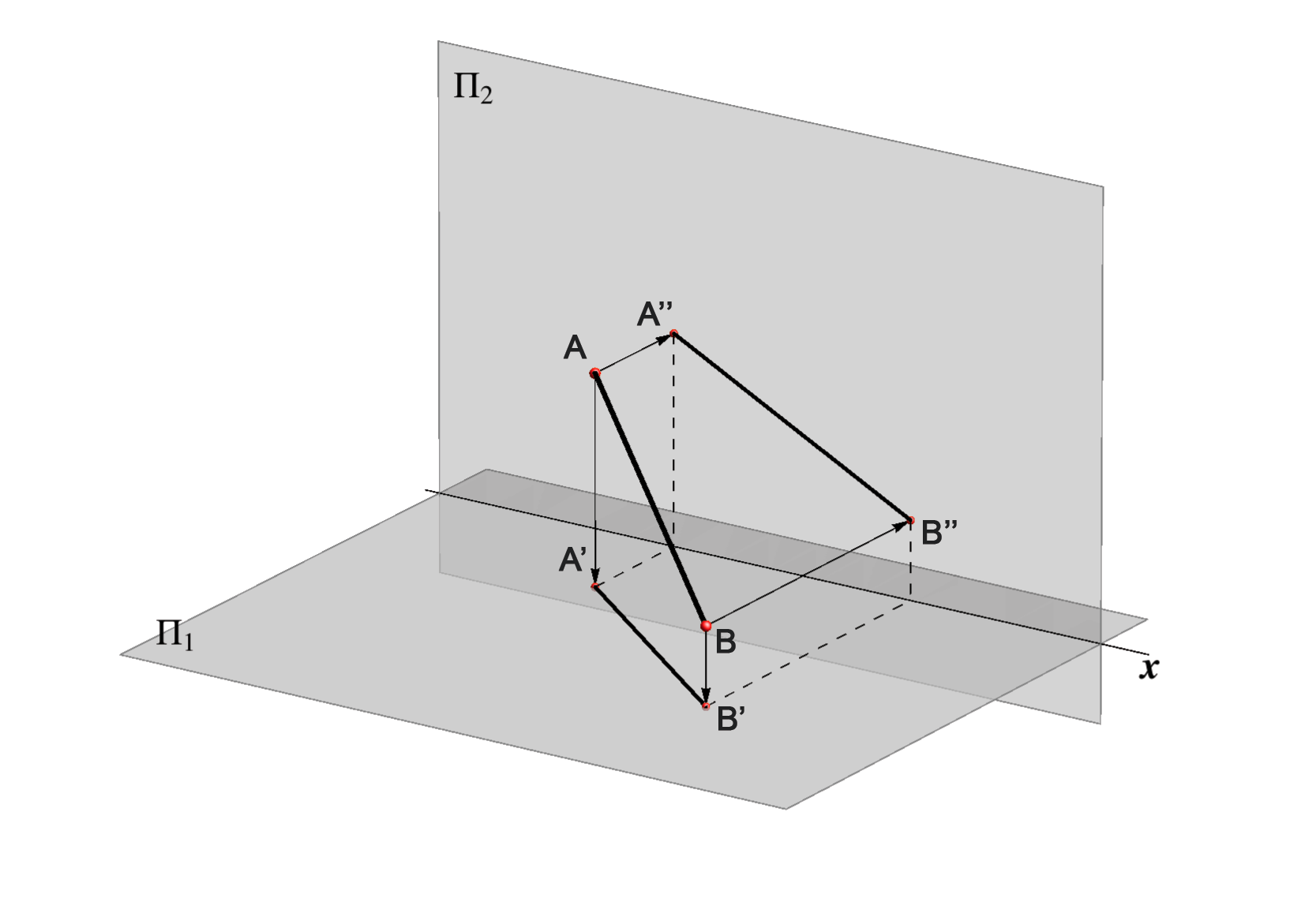If a line segment AB is on an oblique line, then its projections have smaller length, i.e. d(A,B) > d(A',B') & d(A,B) > d(A'',B''). This fact is obtained from the properties of the right trapezoids shown in the picture on the right.It is very important to determine the true length of a line segment AB given with its projections  (A'B',A''B'').

• We rotate the right trapezoid  ABB'A' around the side A'B' for the right angle into the horizontal plane.

Point A corresponds to the point Ao , point B to Bo and the following relation holds: d(A,B) = d(Ao,Bo).
The length of A'Ao equals the z-coordinate of the point A, and the same holds for B, so this trapezoid A'AoB'Bo can easily be constructed in the horizontal plane.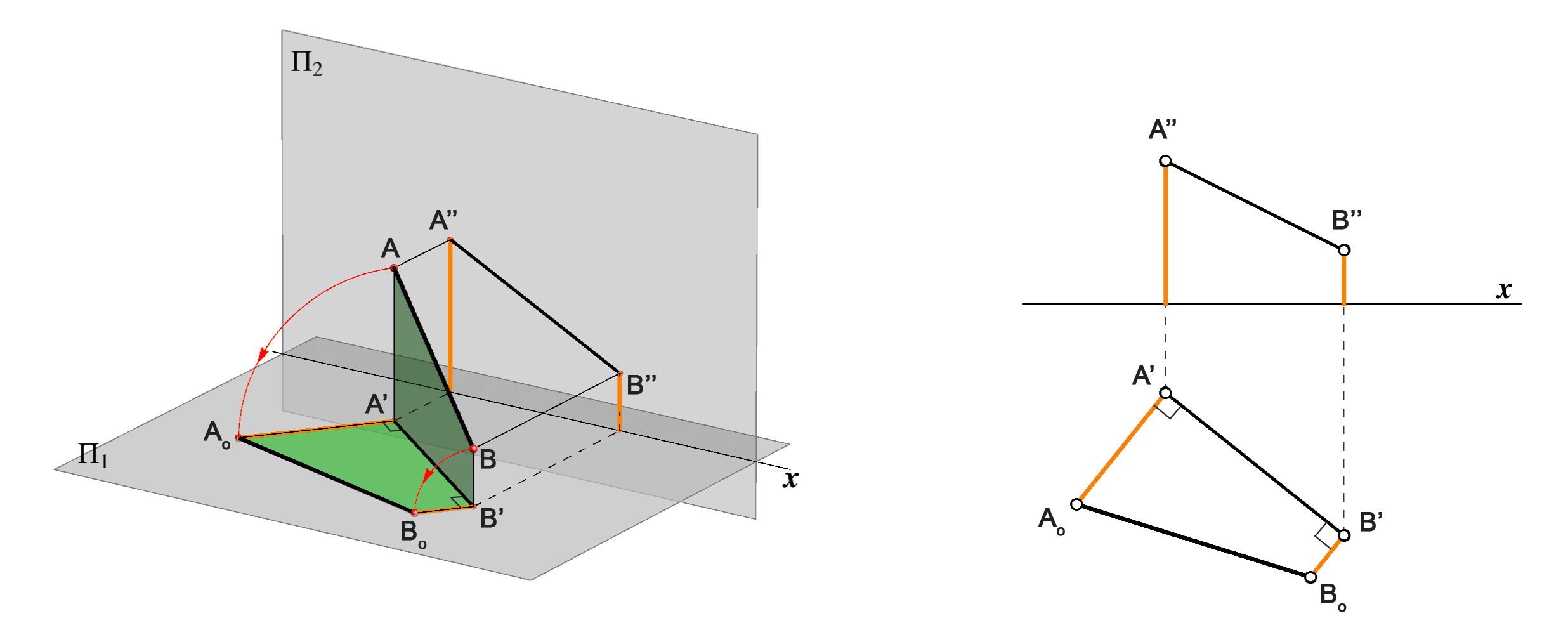Determining the true length of a line segment-1

• If the z-coordinates of points A and B have opposite signs, after the rotation we obtain two right triangles.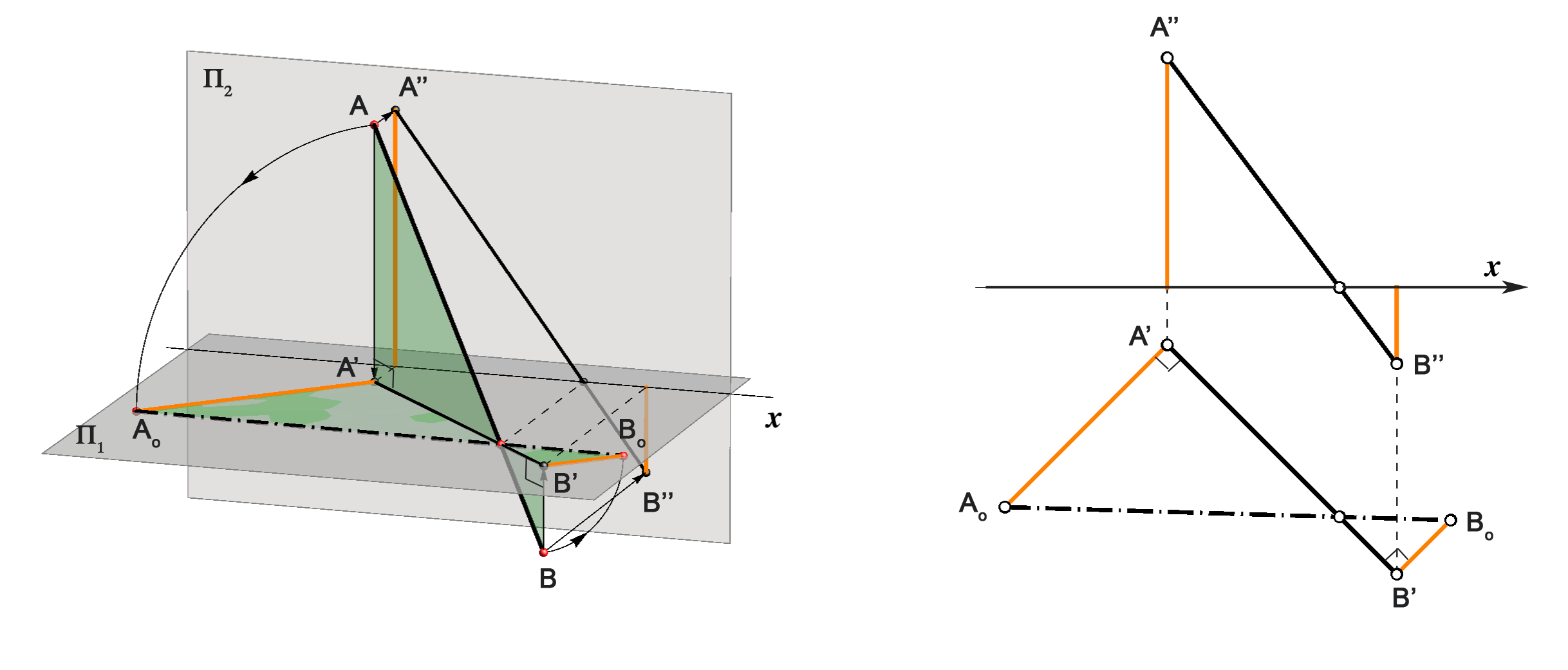We can also rotate the right trapezoid ABB''A''  into the vertical plane. We denote the image of A and B with Ao and Bo.
The length of A''Ao equals the y-coordinate of the point A, the same is valid for the point B.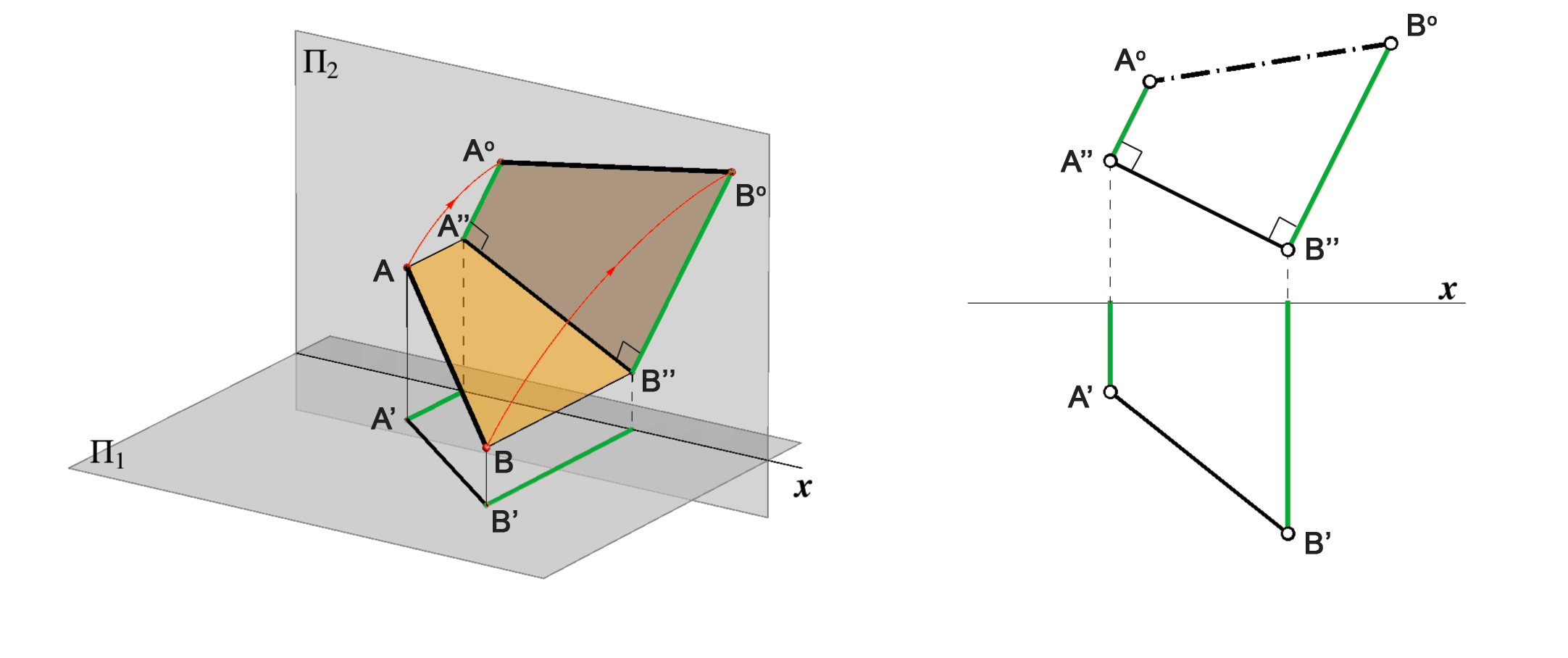Determining the true length of a line segment-2

If the y-coordinates of the points A and B have opposite signs, the image of this trapezoid after the rotation is two right triangles.

Straight line appearing in true length

• Orthogonal projection of a line segment is in true length if and only if the line segment is parallel to the image plane.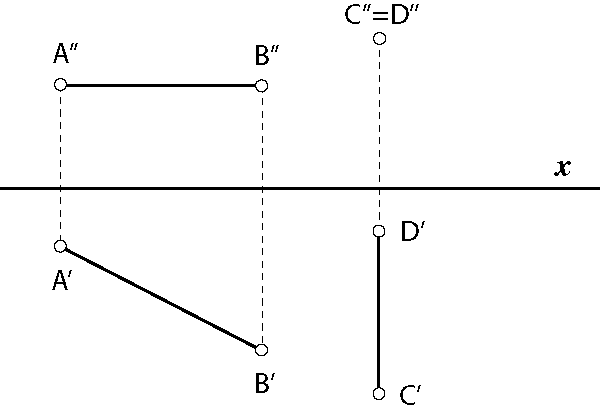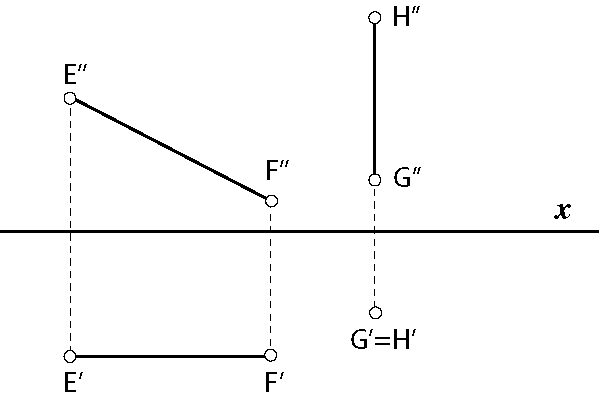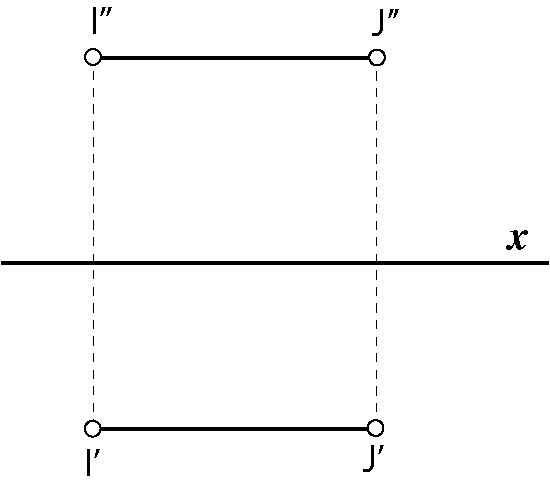Lines AB i CD are parallel to Π1, their horizontal projections are in true length. Lines EF i GH are parallel to Π2, their vertical projections are in true length. Line IJ is parallel to the ground line x, both its projections are in true length.

Created by Sonja Gorjanc 3DGeomTeh - Developing project of the University of Zagreb.
Translated by Helena Halas and Iva Kodrnja.